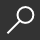## Mathematical Analysis and Numerical Methods for Science and Technology : Volume 2 Functional and Variational Methods by I. N. Sneddon and Robert Dautray and M. Artola

Overview -
These 6 volumes - the result of a 10 year collaboration between the authors, two of France's leading scientists and both distinguished international figures - compile the mathematical knowledge required by researchers in mechanics, physics, engineering, chemistry and other branches of application of mathematics for the theoretical and numerical resolution of physical models on computers. Since the publication in 1924 of the "Methoden der mathematischen Physik" by Courant and Hilbert, there has been no other comprehensive and up-to-date publication presenting the mathematical tools needed in applications of mathematics in directly implementable form. The advent of large computers has in the meantime revolutionised methods of computation and made this gap in the literature intolerable: the objective of the present work is to fill just this gap. Many phenomena in physical mathematics may be modeled by a system of partial differential equations in distributed systems: a model here means a set of equations, which together with given boundary data and, if the phenomenon is evolving in time, initial data, defines the system. The advent of high-speed computers has made it possible for the first time to calculate values from models accurately and rapidly. Researchers and engineers thus have a crucial means of using numerical results to modify and adapt arguments and experiments along the way. Every facet of technical and industrial activity has been affected by these developments. Modeling by distributed systems now also supports work in many areas of physics (plasmas, new materials, astrophysics, geophysics), chemistry and mechanics and is finding increasing use in the life sciences.

local_shippingFor DeliveryIn Stock.
This item is Non-Returnable.
FREE Shipping for Club Members helpNew & Used Marketplace 5 copies from \$103.63

## More About Mathematical Analysis and Numerical Methods for Science and Technology by I. N. Sneddon; Robert Dautray; M. Artola

### Overview

These 6 volumes - the result of a 10 year collaboration between the authors, two of France's leading scientists and both distinguished international figures - compile the mathematical knowledge required by researchers in mechanics, physics, engineering, chemistry and other branches of application of mathematics for the theoretical and numerical resolution of physical models on computers. Since the publication in 1924 of the "Methoden der mathematischen Physik" by Courant and Hilbert, there has been no other comprehensive and up-to-date publication presenting the mathematical tools needed in applications of mathematics in directly implementable form. The advent of large computers has in the meantime revolutionised methods of computation and made this gap in the literature intolerable: the objective of the present work is to fill just this gap. Many phenomena in physical mathematics may be modeled by a system of partial differential equations in distributed systems: a model here means a set of equations, which together with given boundary data and, if the phenomenon is evolving in time, initial data, defines the system. The advent of high-speed computers has made it possible for the first time to calculate values from models accurately and rapidly. Researchers and engineers thus have a crucial means of using numerical results to modify and adapt arguments and experiments along the way. Every facet of technical and industrial activity has been affected by these developments. Modeling by distributed systems now also supports work in many areas of physics (plasmas, new materials, astrophysics, geophysics), chemistry and mechanics and is finding increasing use in the life sciences.

This item is Non-Returnable.

### Details

• ISBN-13: 9783540660989
• ISBN-10: 3540660984
• Publisher: Springer
• Publish Date: November 1999
• Page Count: 590
• Dimensions: 9.21 x 6.14 x 1.23 inches
• Shipping Weight: 1.86 poundsRelated Categories